Scott E Umbaugh, The CRC Press, ISBN: 0-84-932919-1.

Errata File

August 11, 2008

Errors/Changes for the 4th  printing of the book:

Ø Chapter 6, p. 275, the variance (sigma) equations, the ‘1s’ should be ‘i’and ‘j’, respectively:It is:

It should be:Ø Chapter 10, p. 494, Example 10.2.4, the closing bracket on the second line of the equation is in the wrong place, it should be at the end:

It is: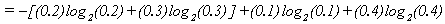It should be: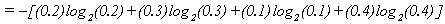January 17, 2007

Errors/Changes for 1st, 2nd, and 3rd (Nov 2006) printing of the book:

Ø Chapter 3,  page 72, middle of page, convolution mask for 1st order hold should be:Note: the zeros in the corner of the original should be ¼

Ø Chapter 3, p. 85, second line of text from the bottom of the page, change “lines” to “edges”:

It is:

“…, corresponding to lines in the vertical,”

It should be:

“…, corresponding to edges in the vertical,”

Ø Chap. 4, p. 140, caption of Figure 4.2.12c:

It is:

“(c) Roberts result, FOM = 0.853”

It should be:

“(c) Roberts result, FOM = 0.498”

Ø Chapter 4, p. 148, figure 4.2.18. In the flowchart the “-90” should be “0”, and the “90” should be “180”, as follows: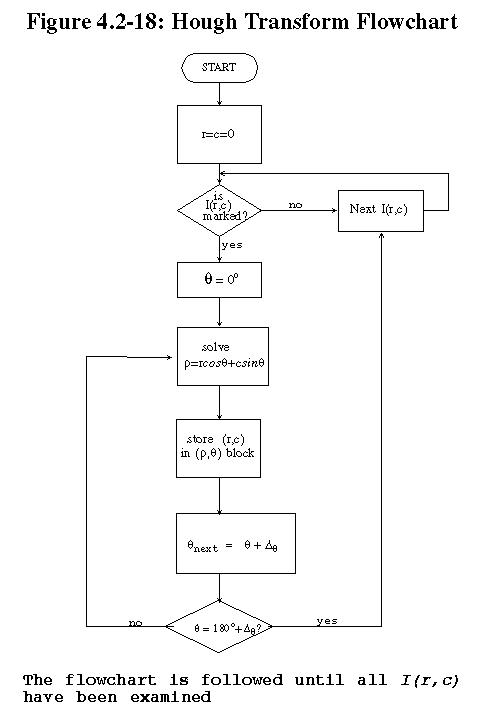Ø Chapter 4, Example 4.3.1, bottom of p. 169, the STRUCTURING ELEMENT is incorrect:

It is:It should be: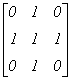Ø Chapter 4, Example 4.3.2, p. 171, the IMAGE is incorrect; 4th  row, 4th column, the ’0’ should be a ‘1’:

It is: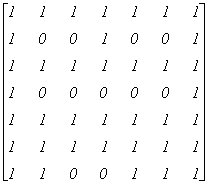It should be:Ø  Chapter 4, Example 4.3.2, p. 171, the RESULT is incorrect; 5th  row, 2th column, the ’0’ should be a ‘1’:

It should be: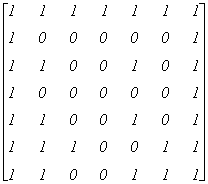Ø Chapter 4, Example 4.3.3, p. 178, middle of the page, “the resultant image” is incorrect:

It is:It should be: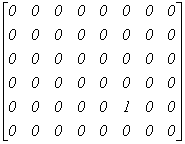Ø Chapter 4, Example 4.3.8, p. 181, middle of the page, “the resultant image” is incorrect: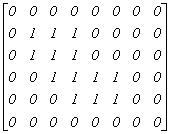It is:

It should be: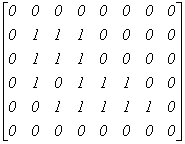Ø Chap 5, p. 204, Fig. 5.1.4. The layout of the figure was changed so that all four of the boxes are across the page – this is wrong; it is critical they are laid out (two-dimensionally) as follows:Ø Chapter 5, p. 207, Figure 5.2.1 caption, (b)-(e):

It is:

“(b)The fundamental. (c) The first harmonic. (d) The second harmonic. (e) Approximation to the sum of the fundamental and the first three harmonics.”

It should be:

“(b)The fundamental, or first harmonic. (c) The second harmonic. (d) The third harmonic. (e) Approximation to the sum of the first three harmonics.”

Ø Chapter 5, p. 209, the equation in the middle of the page, after “The inverse DFT is given by:”, the I(r,c) should be I(c), and the 1/N should not be there, as follows:

It is: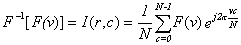It should be:Ø Chapter 5, p. 209, the lower part of the page, change the “R(v)” to “Re(v)”and “I(v)” to “Im(v)” in both the equations and text. This is needed in a total of five “R(v)” and “I(v)” pairs.

It is:

“…the one-dimensional DFT equation can be written as: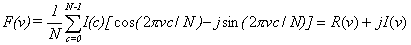In this case, F(v) is also complex, with the real part corresponding to the cosine terms, and the imaginary part corresponding to the sine terms. If we represent a complex spectral component by F(v) = R(v) + jI(v), where R(v) is the real part and I(v) is the imaginary part, then we can define the magnitude and phase of a complex spectral component as: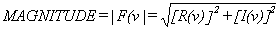and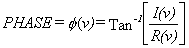It should be:

“…the one-dimensional DFT equation can be written as: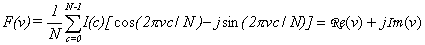In this case, F(v) is also complex, with the real part corresponding to the cosine terms, and the imaginary part corresponding to the sine terms. If we represent a complex spectral component by F(v) = Re(v) + jIm(v), where Re(v) is the real part and Im(v) is the imaginary part, then we can define the magnitude and phase of a complex spectral component as:andØ Chapter 5, p. 210, Figure 5.2.4, change the “R(v)” to “Re(v)”and “I(v)” to “Im(v)” in both the equations and text. Below is the corrected figure:

Figure 5.2.4 Complex Numbers. a) A complex number shown as a vector and expressed in rectangular form,  in terms of the real, Re, and imaginary components, Im, b) a complex number expressed in exponential form in terms of magnitude, M, and angle, θ. Note that θ is measured from the real axis counterclockwise.

a)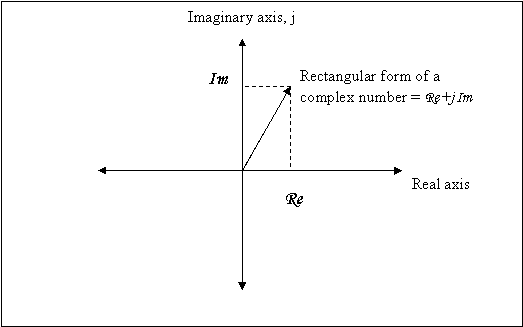b)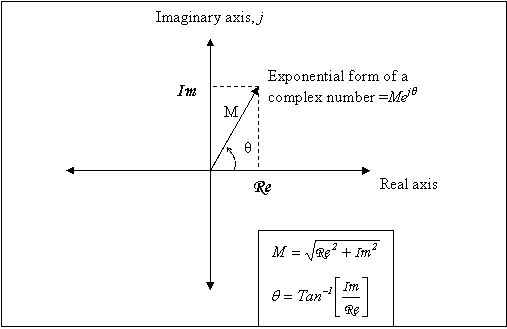Ø Chapter 5, p. 211, Example 5.2.2, the third equation down has an extra “2” in it:

It is as follows:It should be:It is: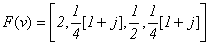It should be: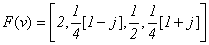Ø Chapter 5, p. 213, top to middle part of the page, change the “R(v)” to “Re(v)”and “I(v)” to “Im(v)” in both the equations and text; there are four “R(v)” and “I(v)” pairs.

It is:

“If we represent a complex spectral component by F(u,v) = R(u,v) + jI(u,v), where R(u,v) is the real part and I(u,v) is the imaginary part, then we can define the magnitude and phase of a complex spectral component as: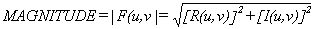And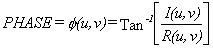It should be:

“If we represent a complex spectral component by F(u,v) = Re(u,v) + jIm(u,v), where Re(u,v) is the real part and Im(u,v) is the imaginary part, then we can define the magnitude and phase of a complex spectral component as: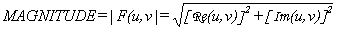andØ    Chapter 5, Section 5.2.2, page 214, second equation on the page. The first “vc” should be “ur”:

It is:It should be: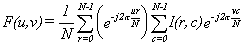Ø Chapter 5, p. 215 under 5.2.3.4 Modulation. The equations are missing the 1/N term in the exponent, they should be: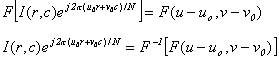Ø Chapter 5, page 217, bottom of page, last part of equation is “v = N”, it should be “v + N”.

It is: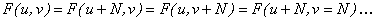It should be: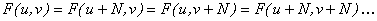Ø Chapter 5, page 247, PERIODICITY equation, last part of equation is “v = N”, it should be “v + N”.

It is: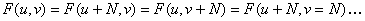It should be:Ø Chap 5, p. 218, Section 5.2.4, first sentence, it says:

“The Fourier spectrum consists of complex floating point numbers, which are stored in CVIPtools as a two band image – one band for the real plane data, and one band for the imaginary plane data.”

It should be:

“The Fourier spectrum consists of complex floating point numbers, which are stored in CVIPtools as in a single band image with a data format of complex. “

Ø Chapter 5, p. 227, Example 5.4.3, the second line, the figure number is wrong.

It is as follows: “image in Figure 2.5.10…”,

It should be: “image in Figure 5.4.3…”

Ø Chapter 5. p. 231, (RGB’s missing) equation after “3. Perform the linear transform on the RGB data…”

It is as follows:It should be:Ø Chapter 5, p. 236, Figure 5.7.6, the images in (d) and (e) need to be swapped.

Ø Chapter 5, p. 236, Figure 5.7.6, the caption is wrong, it should be as follows:

“Figure 5.7.6 Highpass filter functions. a) 1-D ideal highpass filter. b) 1-D nonideal highpass filter. c) 2-D ideal highpass filter for Fourier symmetry, shown as an image. d) 2-D nonideal highpass filter for Fourier symmetry.  e) 2-D ideal highpass filter for cosine and Walsh-Hadamard symmetry. f) 2-D nonideal highpass filter for cosine and Walsh-Hadamard symmetry. Note: for the filters shown as images, white =1, black = 0, and gray values in between represent values between 0 and 1, corresponding to the transition band in nonideal filters.”

Ø Chapter 5, p. 237, Figure 5.7.7, the  caption is wrong, it should be as follows:

“Figure 5.7.7 High frequency emphasis filter functions. a) 1-D ideal high frequency emphasis filter. b) 1-D nonideal high frequency emphasis filter. c) 2-D ideal high frequency emphasis filter for Fourier symmetry, shown as an image. d) 2-D nonideal high frequency emphasis filter for Fourier symmetry.  e) 2-D ideal high frequency emphasis filter for cosine and Walsh-Hadamard symmetry. f) 2-D nonideal high frequency emphasis filter for cosine and Walsh-Hadamard symmetry.

Ø Chapter 5, p. 239, Section 5.8 Wavelet Transform, the second paragraph, third sentence (swap the last “vertical” and “horizontal”).

It is as follows:

“The results consist of one image that has been highpass filtered in both the horizontal and vertical directions, one that has been highpass filtered in the vertical and lowpassed in the horizontal, one that has been highpassed in the vertical and lowpassed in the horizontal, and one that has been lowpass filtered in both directions. “

It should be:

“The results consist of one image that has been highpass filtered in both the horizontal and vertical directions, one that has been highpass filtered in the vertical and lowpassed in the horizontal, one that has been highpassed in the horizontal and lowpassed in the vertical, and one that has been lowpass filtered in both directions. “

Ø Chapter 5, p. 241, Example 5.8.2. The “origin” and its corresponding arrow in the equations are pointing at the wrong coefficient. They should be as follows (need to be moved to the right):Ø Chapter 5, p. 246, under One-dimensional DFT, second line of the FORWARD equation, change the “R(v)” to “Re(v)”and “I(v)” to “Im(v)”.

It is:It should be: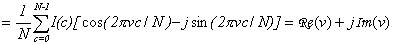Ø Chapter 5, p. 246, under One-dimensional DFT, INVERSE equation, change “I(r,c)” to “I(c)” and remove the “1/N” term:

It is:It should be: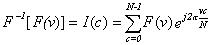Ø Chapter 5, p. 246, under “One-dimensional DFT” change the “R(v)” to “Re(v)”and “I(v)” to “Im(v)” in the MAGNITUDE and PHASE equations.

It is:It should be:Ø Chapter 5, p. 247, middle of page, under MODULATION. The equations are missing the 1/N term in the exponent, they should be:Ø Chapter 5, p. 251, Wavelet Transform, 5th bullet (swap the last “horizontal” and “vertical”):

It is as follows:

“The wavelet results consist of one subsampled image that has been highpass filtered in both the horizontal and vertical directions, one that has been highpass filtered in the vertical and lowpassed in the horizontal, one that has been highpassed in the vertical and lowpassed in the horizontal, and one that has been lowpass filtered in both directions. “

It should be:

“The wavelet results consist of one subsampled image that has been highpass filtered in both the horizontal and vertical directions, one that has been highpass filtered in the vertical and lowpassed in the horizontal, one that has been highpassed in the horizontal and lowpassed in the vertical, and one that has been lowpass filtered in both directions. “

Ø Chapter 6, p. 276, bottom of page, equation for Laws wave, W5   (the last ‘-1’ should be a ‘1’):

It is:

“W5  =  (-1, 2, 0, -2, -1)”

It should be:

“W5  =  (-1, 2, 0, -2, 1)”

Ø Chapter 6, p. 295, middle of page, equation for Laws wave, W5   (the last ‘-1’ should be a ‘1’):

It is:

“W5  =  (-1, 2, 0, -2, -1)”

It should be:

“W5  =  (-1, 2, 0, -2, 1)”

Ø Chapter 8, Section 8.2.3, p. 370, equations for Log-ACE , definition of MAX.

It is:

“…MAX is maximum gray value (e.g. 255)”

It should be:

“…MAX is number of gray values (e.g. 256)”

Ø Chapter 8, Section 8.2.3, p. 370, equations for Exp-ACE , definition of MAX.

It is:

“MAX = maximum gray value (e.g. 255)”

It should be:

“MAX = number of gray values (e.g. 256)”

Ø Chapter 8, p. 391, 8.5 Key Points, GRAY SCALE MODIFICATION, bullet one:

It is as follows: “Gray scale modification is also called gray level scaling or gray level modification

It should be: “Gray scale modification is also called gray level scaling or gray level transformation

Ø Chapter 9, p. 427, equations on bottom that have I(r,c) should be d(r,c):

It is:Ø Chapter 9, p. 429-430 & 465, the subscripts min and max need swapped, in two places.

It is:It should be:Ø Chap. 9, p. 454, Example 9.6.1, the last line:

It is:

“Now, we let I(2,3) = d(39,27)”

It should be:

“Now, we let I(2,3) = d(39,17)”

Ø Chapter 10, p. 494, Example 10.2.4, the closing bracket on the second line of the equation is in the wrong place, it should be at the end:

It is:It should be:Ø Chap. 10, p. 515, middle of page, the first I-tilde should be I-hat

It is as follows:

This error signal is then quantized, such that:It should be:

“This error signal is then quantized, such that: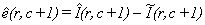Ø Chap. 10, p. 533, Fig. 10.3.24. The images in (d) and (e) need to be swapped (note: it is correct in the color plate).

Ø Chapter 11, p. 562, number 9, the “print_CVIP” line of code has an extra “rdquo;” that needs to be removed.

It is:

print_CVIP("test new function, return value is %d\n",rdquo; test_function(1));

It should be:

print_CVIP("test new function, return value is %d\n",test_function(1));

Ø Chap 12., p. 596, under the morpho function, fifth line (change to “a+b”):

It is as follows:

“boolFUNC> ‑ integer number for the Boolean function (1‑6): 1: 0,  2: !a, 3: ab,  4: a plus;b,

It should be:

“boolFUNC> ‑ integer number for the Boolean function (1‑6): 1: 0,  2: !a, 3: ab,  4: a+b,”

Ø In the index, p. 648, under “Data”, add the following entry:

“structure,  566, 569-573”

Ø In the index, p. 652, under “Image”, add the following:

“structure, 570”

Ø In the index, p. 654, under “Matrix”, add the following:

“structure, 569”

Ø In the index on page 656, the entry for Reflectance function should also have page 382.

It  is:

“Reflectance function, 20, 21, 58”

It should be:

“Reflectance function, 20, 21, 58, 382”